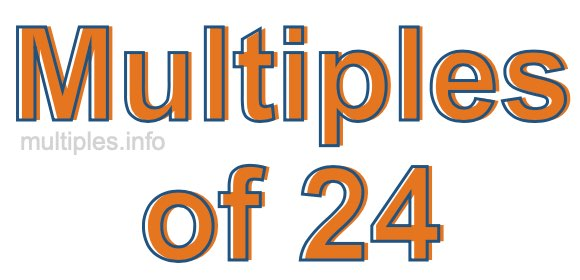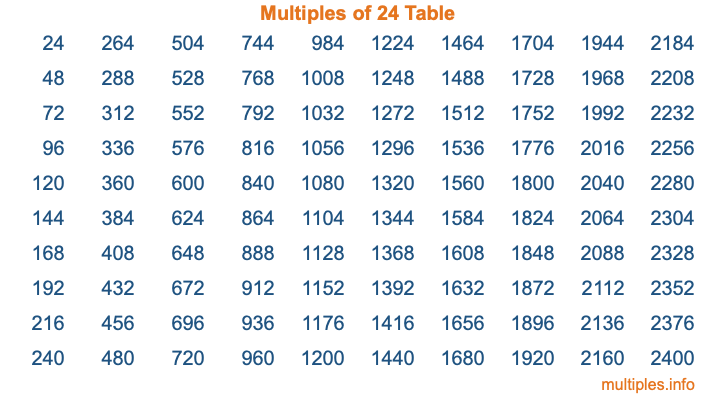Multiples of 24Welcome to the Multiples of 24 page. Here we will first teach you everything you will ever need to know about the multiples of 24, and then give you a study guide summary of everything we taught you to make sure you remember it all. Use this page to look up facts and learn information about the multiples of 24. This page will make you a multiples of twenty-four expert!

Definition of Multiples of 24
Multiples of 24 are all the numbers that when divided by 24 equal an integer. Each of the multiples of 24 are called a multiple. A multiple of 24 is created by multiplying 24 by an integer.

Therefore, to create a list of multiples of 24, you start with 1 multiplied by 24, then 2 multiplied by 24, then 3 multiplied by 24, and so on for as long as you want. Thus, the list of the first five multiples of 24 is 24, 48, 72, 96, and 120. To see a larger list of multiples of 24, see the printable image of Multiples of 24 further down on this page. We also have a category where you can choose any nth multiple of 24.

Multiples of 24 Checker
The Multiples of 24 Checker below checks to see if any number of your choice is a multiple of 24. In other words, it checks to see if there is any number (integer) that when multiplied by 24 will equal your number. To do that, we divide your number by 24. If the the quotient is an integer, then your number is a multiple of 24.

Is  a multiple of 24?

Least Common Multiple of 24 and ...
A Least Common Multiple (LCM) is the lowest multiple that two or more numbers have in common. This is also called the smallest common multiple or lowest common multiple and is useful to know when you are adding our subtracting fractions. Enter one or more numbers below (24 is already entered) to find the LCM.

Check out our LCM Calculator if you need more details about the Least Common Multiple or if you need the LCM for different numbers for adding and subtraction fractions.

nth Multiple of 24
As we stated above, 24 is the first multiple of 24, 48 is the second multiple of 24, 72 is the third multiple of 24, and so on. Enter a number below to find the nth multiple of 24.

th multiple of 24

Multiples of 24 vs Factors of 24
24 is a multiple of 24 and a factor of 24, but that is where the similarities end. All postive multiples of 24 are 24 or greater than 24. All positive factors of 24 are 24 or less than 24.

Below is the beginning list of multiples of 24 and the factors of 24 so you can compare:

Multiples of 24: 24, 48, 72, 96, 120, etc.

Factors of 24: 1, 2, 3, 4, 6, 8, 12, 24

As you can see, the multiples of 24 are all the numbers that you can divide by 24 to get a whole number. The factors of 24, on the other hand, are all the whole numbers that you can multiply by another whole number to get 24.

It's also interesting to note that if a number (x) is a factor of 24, then 24 will also be a multiple of that number (x).

Multiples of 24 vs Divisors of 24
The divisors of 24 are all the integers that 24 can be divided by evenly. Below is a list of the divisors of 24.

Divisors of 24: 1, 2, 3, 4, 6, 8, 12, 24

The interesting thing to note here is that if you take any multiple of 24 and divide it by a divisor of 24, you will see that the quotient is an integer.

Multiples of 24 Table
Below is an image of the first 100 multiples of 24 in a table. The table is in chronological order, column by column. The first column has the first ten multiples of 24, the second column has the next ten multiples of 24, and so on.The Multiples of 24 Table is also referred to as the 24 Times Table or Times Table of 24. You are welcome to print out our table for your studies.

Negative Multiples of 24
Although not often discussed or needed in math, it is worth mentioning that you can make a list of negative multiples of 24 by multiplying 24 by -1, then by -2, then by -3, and so on, to get the following list of negative multiples of 24:

-24, -48, -72, -96, -120, etc.

Multiples of 24 Summary
Below is a summary of important Multiples of 24 facts that we have discussed on this page. To retain the knowledge on this page, we recommend that you read through the summary and explain to yourself or a study partner why they hold true.

There are an infinite number of multiples of 24.

A multiple of 24 divided by 24 will equal a whole number.

24 divided by a factor of 24 equals a divisor of 24.

The nth multiple of 24 is n times 24.

The largest factor of 24 is equal to the first positive multiple of 24.

24 is a multiple of every factor of 24.

24 is a multiple of 24.

A multiple of 24 divided by a divisor of 24 equals an integer.

24 divided by a divisor of 24 equals a factor of 24.

Any integer times 24 will equal a multiple of 24.

Multiples of a Number
Here you can get the multiples of another number, all with the same attention to detail as we did for multiples of 24 on this page.

Multiples of
Multiples of 25
Did you find our page about multiples of twenty-four educational? Do you want more knowledge? Check out the multiples of the next number on our list!